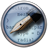# Reusing electrodes in device calculations¶

A typical study of electronic devices with QuantumATK involves systematically studying the influence of various modifications of the device region. The variation can be atomic positions or types of dopants/vacancies in a semiconductor wire, or a different molecule attached to a nanotube, etc. In any case, the electrodes often remain unchanged. One can therefore calculate the electronic structure of the electrodes only once, and then reuse them in other calculations to save computational time.

In this tutorial you will therefore learn how to reuse the devices electrodes for different calculations, thus saving time by only having to calculate the converged state of the electrodes once.

Note

Methodology
An QuantumATK device calculation is usually a two-step process: First the self-consistent states of the electrodes are calculated, and then the actual device calculation is done. Any post-SCF analysis and other operations are done after those two steps.

Reusing the initial electrode calculations is done like this: Starting out from a Python script designed for a normal device calculation as outlined just above, you split that calculation into two scripts: One script that calculates and saves the self-consistent state of the electrodes, and another script that reuses those electrodes and starts directly on the device calculation.

Warning

Calculator settings
It is important that the parameters used for the electrode calculators are the same as those that will be used in the final device calculation, or at least very similar. Otherwise the electrode Fermi level alignment vs. the device average Fermi level may change, which can cause problems for SCF convergence.

Therefore, first make a decision on the numerical accuracy parameters, such as exchange–correlation functional, temperature, mesh cut-off, basis set, etc., and then stick to these for the whole sequence of calculations.

Moreover, it is absolutely necessary that the electrode and device k-point sampling match each other in the A and B directions! You can not use 3 k-points along A in the device but 7 k-points along A in the electrodes.

## Separate scripts for electrodes and device¶

A bit of scripting is required, but mostly copying/pasting existing lines of Python code:

1. Start by setting up the full device calculation as you would normally with some representative central region. Use the Script Generatorfor this task, and make some good chices for the numerical accuracy parameters to be used.

2. Save two copies of the script: electrode.py and device.py.

3. Open electrode.py in the Editorand scroll down to where the device_configuration is defined. It usually looks like illustrated below. Remove those lines.

device_configuration = DeviceConfiguration(
central_region,
[left_electrode, right_electrode]
)


Tip

The script electrode.py is only used to compute the electrodes. You may want to remove the definition of the central region altogether to keep things tidy, but it’s not strictly necessary.

4. Now scroll down towards the end of the script, and remove everything that has to do with the device calculator. That is, remove all lines from the following comment lines to the end of the script:

#----------------------------------------
# Device Calculator
#----------------------------------------

5. Add instead the following lines at the end of the script, and save the script as electrodes.py.

left_electrode.setCalculator(left_electrode_calculator)
left_electrode.update()
nlsave('left_electrode.nc', left_electrode)
right_electrode.setCalculator(right_electrode_calculator)
right_electrode.update()
nlsave('right_electrode.nc', right_electrode)


Note

In the case of identical electrodes, delete the three lines involving the “right_electrode”, and remove the “left” prefix from all the remaining variables:

electrode.setCalculator(electrode_calculator)
electrode.update()
nlsave('electrode.nc', electrode)

6. Run the electrode script, e.g. Job Manager.

7. Now start modifying the script device.py. Starting from the top, remove all the lines involving the left and right electrode configurations, i.e. delete all lines until (but not including)

# -------------------------------------------------------------
# Central region
# -------------------------------------------------------------

8. Instead, add the following lines at the top of the script, which will read the saved electrodes from file:

left_electrode = nlread("left_electrode.nc")


Hint

In the case of identical electrodes, if only a single electrode calculation was executed and saved, in e.g. electrode.nc, use this file for loading both electrodes.

9. It is recommended, but not strictly necessary, to also remove the definition of the electrode calculators, including the lines in the device calculator:

electrode_calculators=
[left_electrode_calculator, right_electrode_calculator],


That’s it! You have successfully separated the electrode and device calculation into two scripts. When the electrode calculation is done, you can run device.py, and see that it completely bypasses the electrode calculation.

Tip

What’s next?
For each new central region geometry to be computed with these electrodes, just replace the definition of the central_region in device.py, and run the device calculation. Make sure to check the device parameters such as bias voltage, etc.

Alternatively, you can apply steps 7–9 above to any complete device calculation script, keeping the points about the numerical accuracy parameters in mind.